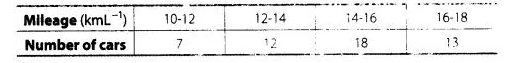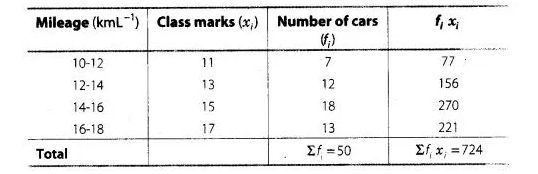# The mileage (km per litre) of 50 cars of

Question:

The mileage (km per litre) of 50 cars of the same model was tested by a manufacturer and details are tabulated as given belowFind the mean mileage.

The manufacturer claimed that the mileage of the model was 16 kmL -1.

Do you agree with this claim?

Solution:Here, $\Sigma f_{i}=50$

and $\Sigma f_{i} x_{i}=724$

$\therefore$ Mean $\bar{x}=\frac{\sum f_{i} x_{i}}{\sum f_{j}}$

$=\frac{724}{50}=14.48$

Hence, mean mileage is $14.48 \mathrm{kmL}^{-1}$.

No, the manufacturer is claiming mileage $1.52 \mathrm{kmh}^{-1}$ more than average mileage.